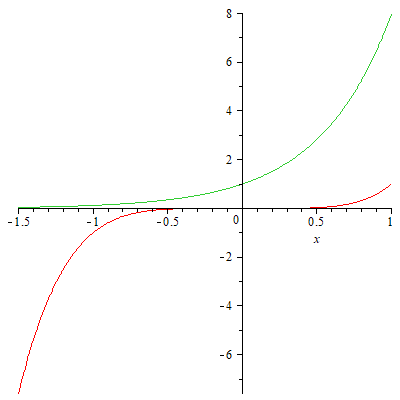Very well, this is really more of a mathematics than a physics post, but so what. Besides, equations of this nature do pop up in physics problems from time to time.

Question (variants of which often appear on Quora): How do you solve the equation, $x^a=b^x$?

Equations of the type

$$x^a=b^x$$

do not usually have solutions in terms of elementary functions, but they can be solved with the help of Lambert's W-function.

The W-function is defined as the solution to the equation

$$W(z)e^{W(z)} = z.$$

Which means that any equation that can be brought to the form $x e^x = {\rm const.}$ can be solved using $W(z).$

So let's do a bit of algebra:

\begin{align*} x^a &= b^x,\\ a\ln x &= x\ln b,\\ (1/x)\ln x &= (1/a)\ln b,\\ (1/x)\ln(1/x) &= -(1/a)\ln b. \end{align*}

Now let $y=\ln(1/x),$ so that $x=1/e^y.$ Then,

$$ye^y = -(1/a)\ln b,$$

so we can now solve for $y$ in terms of the W-function:

$$y=W(-(\ln b)/a).$$

We also note that $e^{W(z)}=z/W(z),$ which follows from the definition of the W-function. With this we get, for $x,$

$$x=\dfrac{1}{e^y}=-\dfrac{a}{\ln b}W\left(-\dfrac{\ln b}{a}\right).$$

This is the general solution.

Here is one specific example: $x^5=8^x.$ So let $a=5$ and $b=8.$ Then we have

$$x=-\dfrac{5}{\ln 8}W\left(-\dfrac{\ln 8}{5}\right)\simeq 2.207-1.183i.$$

Yes, that is a complex number. This particular equation has no real solution. This can be seen easily if we plot the curves $x^5$ (red) and $8^x$ (green), as we can see that they do not intersect anywhere:Additionally, the solution can also be extended to equations of the form

$$cx^a=b^x.$$

These equations can be brought to the previous form as follows:

\begin{align*} cx^a &= b^x\\ (c^{1/a}x)^a &= b^x\\ (c^{1/a}x)^a &= b^{c^{-1/a} c^{1/a} x}\\ (c^{1/a}x)^a &= (b^{c^{-1/a}})^{c^{1/a} x} \end{align*}

Now let
\begin{align*} X &= c^{1/a}x,\\ B &= b^{c^{-1/a}}, \end{align*}

and the equation becomes

$$X^a = B^X,$$

which we already know how to solve:

$$X=-\dfrac{a}{\ln B}W\left(-\dfrac{\ln B}{a}\right).$$

Substituting $A$ and $X$ and noting that $\ln B=c^{-1/a}\ln b$, we get

\begin{align*} c^{1/a}x &= -\dfrac{a}{c^{-1/a} \ln b} W\left(-\dfrac{c^{-1/a} \ln b}{a}\right),\\ x &= -\dfrac{a}{\ln b}W\left(-\dfrac{\ln b}{ac^{1/a}}\right). \end{align*}

One additional caveat concerns the ambiguity arising from the the properties of exponentiation. For instance, the equation $2^x=x^2$ has three real solutions, but the above formalism would yield only two of them (and we get those two because the W-function itself has multiple real values for part of its real domain.) To obtain the third solution, we must recognize that $x^2=(-x)^2$, so we must also solve $2^{-y}=y^2$, i.e., $(1/2)^y=y^2$, with $x=-y$. This yields the third real solution, at $x\simeq -0.766665$.

And, of course, there are infinitely many complex solutions, corresponding to the various branches of the W-function.